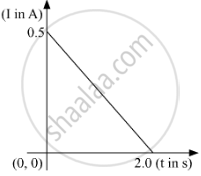# When a conducting loop of resistance 10 Ω and area 10 cm2 is removed from an external magnetic field acting normally, the variation of induced current-I in the loop with time t is as - Physics

Short Note

When a conducting loop of resistance 10 Ω and area 10 cm2 is removed from an external magnetic field acting normally, the variation of induced current-I in the loop with time t is as shown in the figure.
Find the
(a) total charge passed through the loop.
(b) change in magnetic flux through the loop
(c) magnitude of the field applied#### Solution

I = ("dq")/("dt") ⇒ "dq = "I"d"t"

Hence area under the I-t curve gives charge flown.
Area of the I-t curve (as given in the question) = 1/2 xx 2 xx 1/2 = 0.5
Total charge passed through the loop = 0.5 C

Now we know
Δ"Q" = (Δvarphi)/"R"
Δvarphi = Δ"Q" xx "R" = 1/2 xx 10Omega = 5  "Wb"

Charge in magnetic flux through the loop= 5 Wb
Δvarphi = "B"  (Δ"A")
5 = "B"  (0.001)
"B" = 5000 "T"
The magnitude of the field applied = 5000 T

Concept: Magnetic Flux
Is there an error in this question or solution?
2019-2020 (February) Delhi (Set 2)

Share